Tamilnadu State Board New Syllabus Samacheer Kalvi 12th Maths Guide Pdf Chapter 7 Applications of Differential Calculus Ex 7.1 Textbook Questions and Answers, Notes.

## Tamilnadu Samacheer Kalvi 12th Maths Solutions Chapter 7 Applications of Differential Calculus Ex 7.1

Question 1.
A particle moves along a straight line in such a way that after t seconds its distance from the origin is s = 2t² + 3t metres.
(i) Find the average velocity between t = 3 and t = 6 seconds.
(ii) Find the instantaneous velocities at t = 3 and t = 6 seconds.
Solution:
s = 2t² + 3t
(i) Average velocity between t = 3 and t = 6 seconds
Now s(t) = 2t² + 3t
Average velocity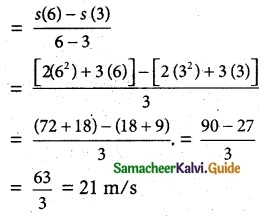(ii) f(t) = 2t2 + 3t
f'(t) = 4t + 3
f'(3) = 4(3) + 3 = 15
f'(6) = 4(3) + 3 = 15Question 2.
A camera is accidentally knocked off an edge of a cliff 400 ft high. The camera falls a distance of s = 16t² in t seconds.
(i) How long does the camera fall before it hits the ground?
(ii) What is the average velocity with which the camera falls during the last 2 seconds?
(iii) What is the instantaneous velocity of the camera when it hits the ground?
Solution:
(i) The camera falls a distance of s = 16t² in t sec.
s = 400 ft
∴ 16t² =400
t² = $$\frac { 400 }{ 16 }$$ = 25
t = 5 sec
∴ Camera falls for 5 sec before it hits the ground.

(ii) In 5 sec camera falls 400 ft (given)
∴ Average velocity in 2 sec
= $$\frac { s(5)-s(3) }{ 5-3 }$$
= $$\frac { 16(5^2)-16(3^2) }{ 2 }$$
= $$\frac { 400-144 }{ 2 }$$
= $$\frac { 256 }{ 2 }$$
= 128 ft/sec

(iii) f(t) = 16t2
f'(t) = 32t
f'(t) at t = 5 = 32(5)
= 160 ft/sec

Question 3.
A particle moves along a line according to the law s(t) = 2t³ – 9t² + 12t – 4, where t ≥ 0.
(i) At what times the particle changes direction?
(ii) Find the total distance travelled by the particle in the first 4 seconds.
(iii) Find the particle’s acceleration each time the velocity is zero.
Solution:
s (t) = 2t³ – 9t² + 12t – 4, t ≥ 0
velocity v = $$\frac { ds }{ dt }$$ = 6t² – 18t + 12
When the particle changes its direction, v = 0
6t² – 18t + 12 = 0 (÷6)
t² – 3t + 2 = 0
(t – 2) (t – 1) = 0
t = 1, 2
∴ When time t = 1 sec and t = 2 sec, the particle changes its direction.(ii) The distance travelled in the first 4 seconds is
|s(0) – s(1)| + |s(1) – s(2)| + |s(2) – s(3)| + |s(3) – s(4)|
Here, s(t) = 2t3 – 9t2 + 12t – 4
s(0) = -4
s(1) = 1
s(2) = 0
s(3) = 5
and s(4) = 28
∴ Distance travelled in the first 4 seconds
= |-4 – 1| + |1 – 0| + |0 – 5| + |5 – 28|
= 5 + 1 + 5 + 23 = 34 m

(iii) s (t) = 2t³ – 9t² + 12t – 4
velocity v = $$\frac { ds }{ dt }$$ = 6t² – 18t + 12
v = 0 ⇒ 6(t² – 3t + 2) = 0 ⇒ t = 1, 2
Acceleration = $$\frac { d^2s }{ dt^2 }$$ = 12t – 18
at t = 1, Acceleration = 12(1) – 18 = -6m/sec²
at t = 2, Acceleration = 12 (2) – 18 = 6 m/sec²

Question 4.
If the volume of a cube of side length x is v = x³. Find the rate of change of the volume with respect to x when x = 5 units.
Solution:
volume of a cube v = x³
Rate of change $$\frac { dv }{ dx }$$ = 3x²
When x = 5 units, $$\frac { dv }{ dx }$$ = 3(5)² = 3(25) = 75 units.Question 5.
If the mass m(x) (in kilograms) of a thin rod of length x (in metres) is given by, m(x) = $$\sqrt { 3x }$$ then what is the rate of change of mass with respect to the length when it is x = 3 and x = 27 metres.
Solution: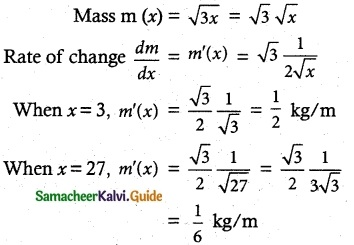Question 6.
A stone is dropped into a pond causing ripples in the from of concentric circles. The radius r of the outer ripple is increasing at a constant rate of 2 cm per second. When the radius is 5 cm find the rate of changing of the total area of the disturbed water?
Solution:
radius = r, Rate of changes of radius $$\frac { dr }{ dt }$$ = 2 and
given r = 5 cm
Area of circle A = πr²
Differentiating w.r.t ‘t’,
$$\frac { dA }{ dt }$$= 2πr$$\frac { dr }{ dt }$$
= 2π (5) (2)
= 20 π
∴ Area of circle (ripple) is increasing at the rate of 20 π cm²/sec.Question 7.
A beacon makes one revolution every 10 seconds. It is located on a ship which is anchored 5 km from a straight shore line. How fast is the beam moving along the shoreline when it makes an angle of 45° with the shore?
Solution: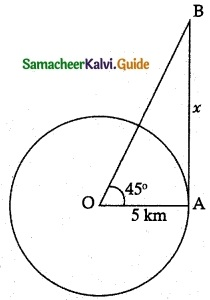Time for one revolution = 10 sec
Now, angular velocity $$\frac { dv }{ dt }$$ = $$\frac { 2π }{ 10 }$$ = $$\frac { π }{ 5 }$$
From the figure, tan 45° = $$\frac { AB }{ OA }$$
1 = $$\frac { x }{ 5 }$$ ⇒ x = 5
Again, tan θ = $$\frac { x }{ 5 }$$
x = 5 tan θ
Differentiating w.r.t. ‘t’
$$\frac { dx }{ dt }$$ = 5 sec² θ $$\frac { dθ }{ dt }$$
= 5 sec² (45°) ($$\frac { π }{ 5 }$$)
= (√2)² π = 2π
∴ The beam is moving at the rate of 2π km/sec.

Question 8.
A conical water tank with a vertex down of 12 metres height has a radius of 5 metres at the top. If water flows into the tank at a rate of 10 cubic m/min, how fast is the depth of the water increases when the water is 8 metres deep?
Solution: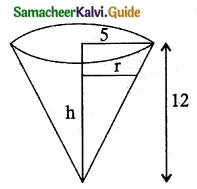From the figure $$\frac { r}{ h }$$ = $$\frac { 5 }{ 12 }$$
r = $$\frac { 5h }{ 12 }$$
given rate of change of volume $$\frac { dV }{ dt }$$ = 10
When h = 8 to find $$\frac { dh }{ dt }$$
Volume of cone V = $$\frac { 1 }{ 3 }$$ πr² h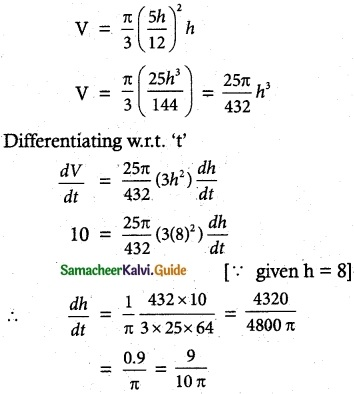The depth of the water increasing at the rate of $$\frac { 9 }{ 10π }$$ m/minQuestion 9.
A ladder 17 metre long is leaning against the wall. The base of the ladder is pulled away from the wall at a rate of 5 m/s. When the base of the ladder is 8 metres from the wall.
(i) How fast is the top of the ladder moving down the wall?
(ii) At what rate, the area of the triangle formed by the ladder, wall, and floor is changing?
Solution: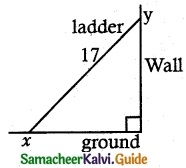Let the height of the wall where the ladder touches are ‘y’ m.
The bottom of the ladder is at a distance of ‘x’ m from the wall.
Given x = 8, $$\frac { dx }{ dt }$$ = 5
x² + y² = 17²
(Pythagoras Theorem)
y² = 17² – x² = 289 – 64 = 225
∴ y = 15
Differentiating w.r.t. ‘t’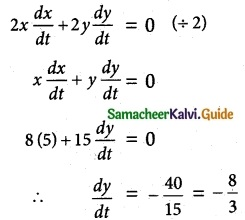(i) The top of the ladder is moving down the wall at the rate of $$\frac { 8 }{ 3 }$$ m/sec
(ii) Area of triangle formed by the ladder, wall and the floor is A = $$\frac { 1 }{ 2 }$$ xy
differentiating w.r.t. ‘t’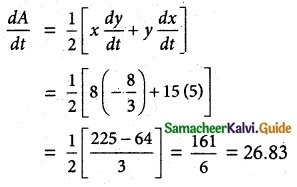∴ Area of the triangle is increasing at the rate of 26.83 m²/sec.

Question 10.
A police jeep, approaching an orthogonal intersection from the northern direction, is chasing a speeding car that has turned and moving straight east. When the jeep is 0.6 km north of the intersection and the car is 0.8 km to the east. The police determine with a radar that the distance between them and the car is increasing at 20 km/hr. If the jeep is moving at 60 km/hr at the instant of measurement, what is the speed of the car?
Solution: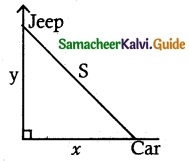given x = 0.8, y = 0.6, $$\frac { dy }{ dt }$$ = -60
and $$\frac { ds }{ dt }$$ = 20
from the figure
S² = x² + y²,
S² = (0.8)² + (0.6)² = 0.64 + 0.36 = 1
S² = 1 ⇒ S = 1
S² = x² + y²,
Differentiating w.r.t. ‘t’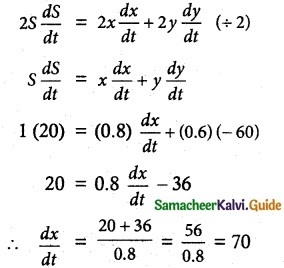∴ Speed of the car is 70 km/hr.